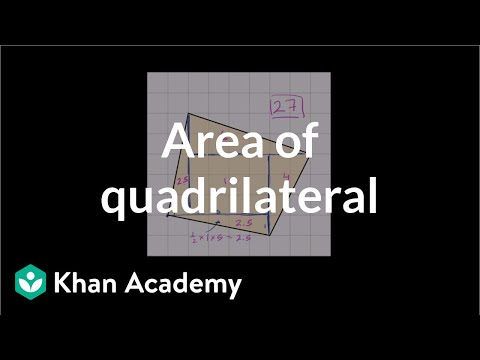Video

# Area of a parallelogram (Full video)

Description: Understand why the formula for the area of a parallelogram is base times height, just like the formula for the area of a rectangle. - If we have a rectangle with base length b and height length h, we know how to figure out its area. Its area is just going to be the base, is going to be the base times the height. So in a situation like this when you have a parallelogram, you know its base and its height, what do we think its area is going to be?

### Other videos you might be interested in### Area of a quadrilateral on a grid (Full video)